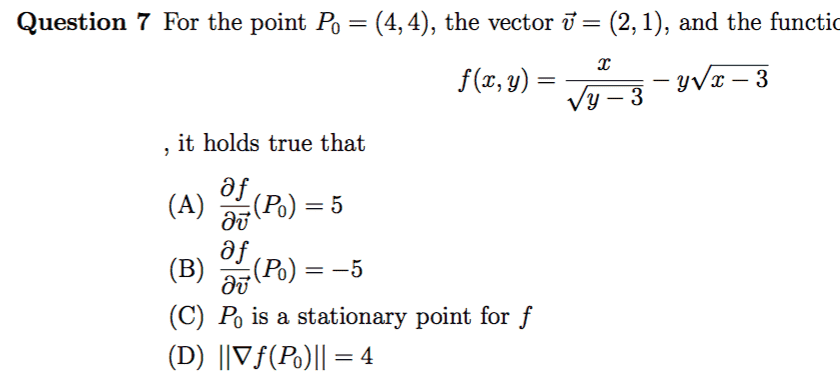# Directional derivative

DottZakapa
Member has been reminded to show some effort from his side.i compute the partial derivative, the vector that i have to use the one in the text or
w=(2/(5^(1/2)), 1/(5^(1/2)))

using the last one i get minus square root of five , if i don't divide by the norm the answer should be B.
i don't understand what D means

Homework Helper
Gold Member
2022 Award
View attachment 255446
i compute the partial derivative, the vector that i have to use the one in the text or
w=(2/(5^(1/2)), 1/(5^(1/2)))

using the last one i get minus square root of five , if i don't divide by the norm the answer should be B.
i don't understand what D means

A direction derivative should be taken wrt a unit vector, so ##-\sqrt{5}## looks right. Unless ##\frac{\partial f}{\partial \vec v}## means something else?

The expression in ##D## is the magnitude of the gradient of ##f## at that point. Sometimes ##||u||## is used instead of ##|u|## for the "norm" or magnitiude of vector.

A direction derivative should be taken wrt a unit vector, so ##-\sqrt{5}## looks right. Unless ##\frac{\partial f}{\partial \vec v}## means something else?

The expression in ##D## is the magnitude of the gradient of ##f## at that point. Sometimes ##||u||## is used instead of ##|u|## for the "norm" or magnitiude of vector.

There are authors who do not ask for this. For example Munkres in "Analysis on manifolds" who defines the directional derivative for any non-zero vector.

Also, I believe it is better to use ##\Vert v \Vert## (Use \Vert v \Vert as tex command) instead of ##|| v ||## (formats a bit more nicely imo).

DottZakapa
so according to the text what shall i consider ?

so according to the text what shall i consider ?

•